# Lab 3: Convolution

Github Classroom assignment

Please put your answers to written questions in this lab, if any, in a Markdown file named README.md in your lab repo.

## Introduction

Welcome to lab 3! This lab is designed to help you get started with Project 2: Filter.

During this lab, you'll learn about digital image processing, particularly convolution. The lectures covering this topic can be quite dense, but you can rest assured that the programming you'll be doing is nowhere near as complicated.

### Objectives

1. Implement basic, per-pixel processing effects,
2. Learn about kernels and convolution, and
3. Begin using convolution to implement more interesting effects.

## Conceptual Background

Broadly, digital image processing is the processing of images through algorithms in order to modify, enhance, or extract useful information from them. These cover a wide range of applications, including noise removal, feature extraction, image compression, and image enhancement.

In this lab, we will be doing some simple image manipulation. We'll also get to explore convolution!. Before we get into that, though, here is some optional information which might help contextualize what was taught in lecture:

Digital image processing as signal processing

In lecture, you might've heard about how digital image processing is really just a form of signal processing. This is because a digital image can be seen as a discrete 2D signal—specifically, one which maps a 2D coordinate to a value which tells us the color of the image at that point.

What exactly constitutes a signal is not something we can easily get into here. If you're interested to find out more, do your own research, approach a TA, or ask the professor!

Spatial domain vs. frequency domain

In lecture, you might also have heard about the "spatial" and "frequency" domains. Let's recap:

Mathematically, an image can be described by the function , where describes a coordinate in the 2D space of the image. The value of the function at a given pixel's coordinate tells us the color of that pixel.

Since our input to is a coordinate in space, we can think of as being the image's spatial domain representation.

What other representations can there be? Well, can be transformed into , the image's frequency domain representation, via the Fourier transform. Unlike , which describes the color of each point (defined by its coordinates), describes the amplitude and phase of each 2D sinusoid (also defined by its coordinates, in some way) needed to perfectly reconstruct the image. Indeed, can be transformed back into via the inverse Fourier transform.

Confused? Don't worry:

In this lab, we will only be working in the spatial domain.

That said, simply knowing that you can think about images (i.e. signals) in the frequency domain can be quite valuable for a deeper understanding of certain phenomena or effects, such as aliasing, ringing artifacts, and, of course, convolution.

## Getting Started

### GUI Elements

If you haven't done so, accept the Github Classroom assignment, clone the generated repository, and run the application in Qt Creator. A window that looks like this should appear:Figure 1: The application window, with no image or filter selected.

These buttons are fairly self-explanatory, but we'll go through them anyway:

• The Choose Image button allow you to select any image file from your computer to upload to the canvas. We have provided you with some images in the resources directory.
• The Apply Filter button applies the currently-selected filter to the image. Currently, this does nothing because you have neither implemented, nor selected, any filters.
• The Revert to Original Image button reverts the image to its original state.

Interested in how GUI elements like these are set up? Feel free to take a look at mainwindow.h and mainwindow.cpp in our stencil code!

### Command Line Arguments

We will use command line arguments to specify the type of the filter we wish to apply to the image. The available filter types are enumerated below.

Filter types (use these as your command line arguments):

• grayscale
• invert
• identity
• shiftLeft
• shiftRight
Reminder: how to set command line arguments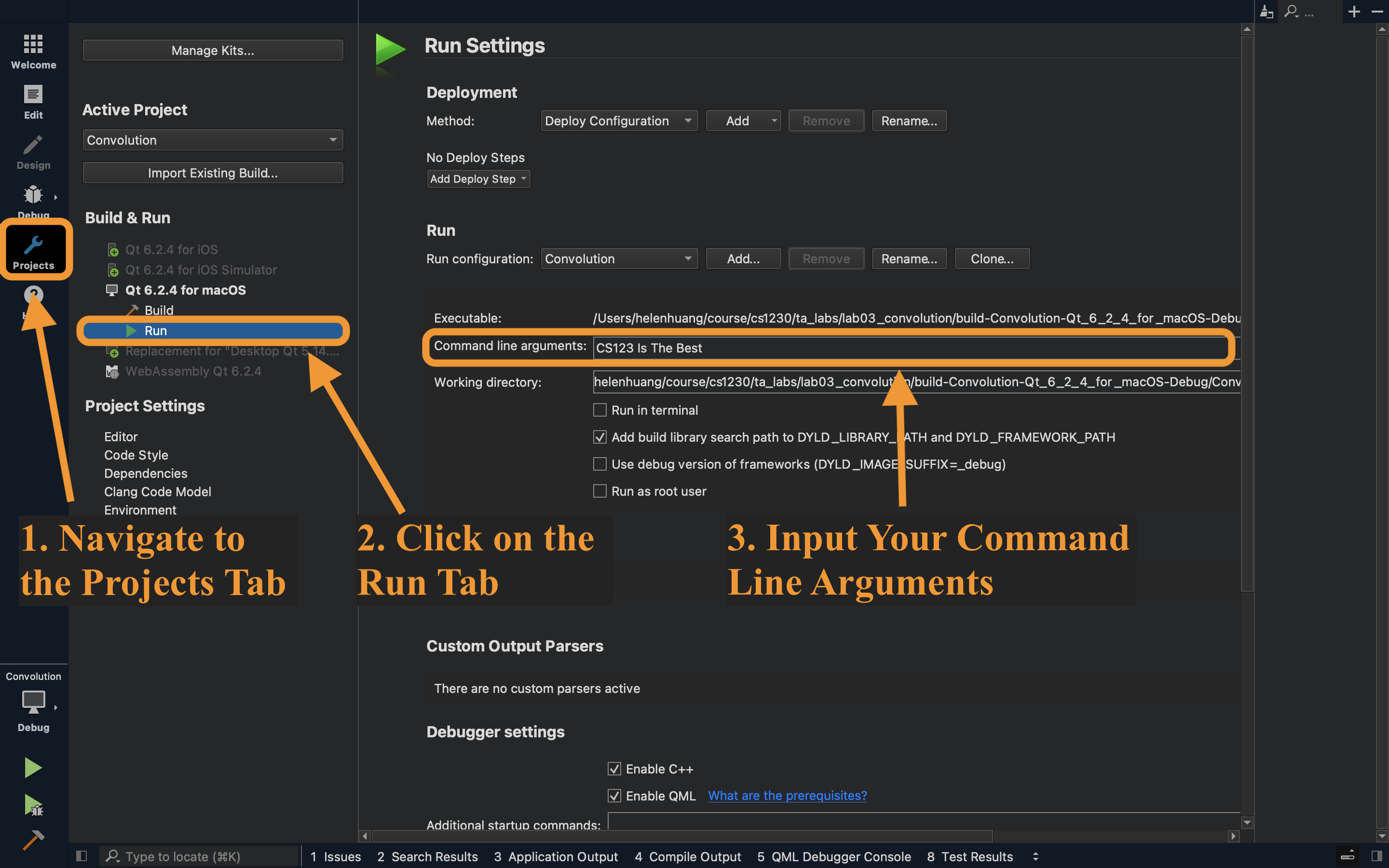Figure 2: Qt view of the command line input

### Stencil Code

Take a look through the provided stencil code. In this lab, you will be working with the following files: canvas2d.h, canvas2d.cpp, the files in the filters directory, and settings.h (read-only).

The Canvas2D class is responsible for storing and manipulating the 2D canvas that will be displayed by the application window. This class has a applyFilter() method which is called whenever the Apply Filter button is clicked.

The filters directory contains several filterXYZ.cpp files, each of which implements a filterXYZ() function. These will be called by applyFilter() to do specific things, such as shifting the image or inverting its colors. The helper functions in filterutils are used to assist in some of these operations.

Notice that the filterXYZ() functions are actually methods of the Canvas2D class; it's perfectly valid, though less common, to implement functions declared in a .h file in one or more differently-named .cpp files.

The settings global object contains information about the specific filter type to be applied to the image. You've already seen a similar object used to specify brush settings in Project 1: Brush.

Let's set up the applyFilter() function so that it applies the appropriate filter when the Apply Filter button is clicked.

In applyFilter(), call the appropriate filter functions depending on the filter type, settings.filterType.

settings.filterType is a FilterType enum, which is defined in settings.h. In C++, you can access enum values by writing: EnumName::EnumValue.

• The four filter functions are declared in canvas2d.h.
• From here, you should notice that filterShift() expects a shiftDirection argument. This must be a ShiftDirection enum value.
• We suggest using a switch statement to keep your code clean and readable!

## Grayscale Filter

The first filter we will implement is the grayscale filter.

This is a "per-pixel" filter—in other words, the final value of each pixel depends only on its original value, and is unaffected by neighboring pixels.

Check out our incomplete implementation of filterGray(), in which we iterate through every pixel in the image.

Within the loop, call the rgbaToGray() function on the currentPixel, then store the result in a temporary variable.

Next, implement rgbaToGray(). This function should return the gray value of a pixel by computing a weighted sum of its red, green, and blue components.

There is no single method to map RGB values to grayscale intensity. We have listed some methods that you can use below:

1. The luma method calculates a weighted sum between the three color channels using percentages that account for the human perceptual system (we recommend this):

2. The average method computes the average between the three color channels:

3. The lightness method, which will desaturate the image, averages the least prominent and most prominent values:

Update the color of the current pixel using the grayscale intensity you stored in task 2.

Running and Testing:

Pass in grayscale as your command line argument and click Run.

Choose an image from your computer (we provided images in the resources/ folder) and click the Apply Filter button.

Your filter should produce the following output:

## Invert Filter

It only takes a little more effort to implement a filter which inverts an image's colors, producing a 'negative' effect. This method works by inverting each color channel of every pixel, which can be done by subtracting its value from the maximum value (255).

In the filterInvert() function, invert each color channel of the currentPixel.

• If you take the inversion of the inverted pixel data, you should end up with the original image! Test this out by simply double-filtering an image with the invert filter.

Running and Testing:

Pass in invert as your command line argument.

Your filter should produce the following output: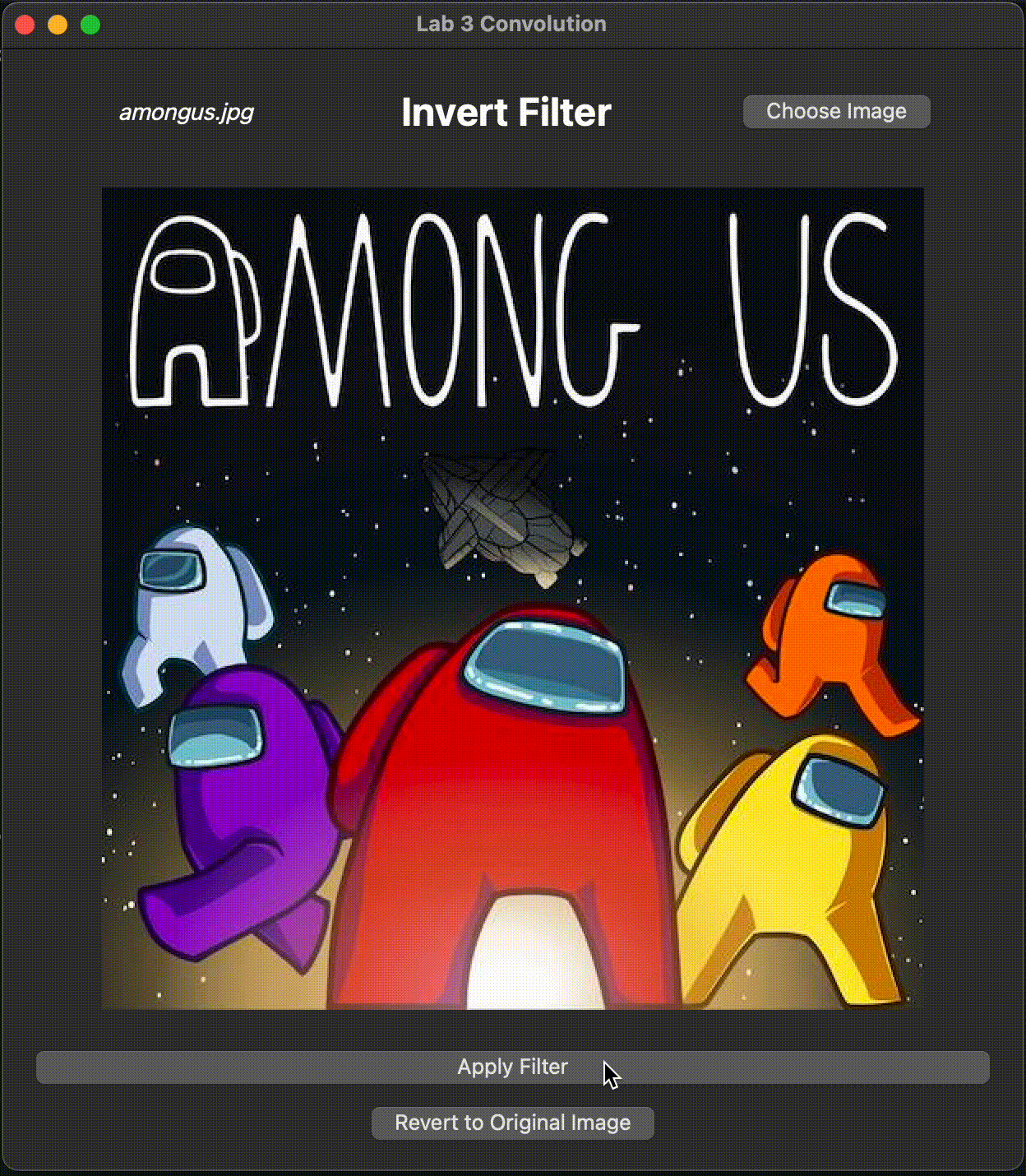Figure 4: A color inversion filter being applied to an image

## Convolution

Thus far, we've only seen filters that deal with each pixel independently. We will now introduce the concept of convolution, which allows us to apply operations to a pixel while taking into account its neighbors.

### Understanding the Process

Generally speaking, convolution is an operation which takes two input signals, and produces an output signal. In digital image processing, we use discrete 2D convolution. This takes in an image and kernel*, and produces an output image.

* A kernel is simply a 2D matrix of values, not unlike an image. And, since convolution is commutative, the distinction between "image" and "kernel" is merely an arbitrary one.

For our purposes, in CS 1230, the steps involved in image convolution as follows:

2. Prepare an output image with the same size as your input image.
3. For each position in this output image:
• Do an element-wise multiplication between:
• The flipped kernel, and
• The region of the image centered on that position, with the same shape as the flipped kernel.
• Then, compute the sum of the elements of the resulting matrix.
• Finally, set the value of the output image at that position to this sum.
Why do we need to "flip" the kernel?

If we were didn't flip the kernel, we would technically be implementing correlation.

Let's look at an example below.

### Worked Example

For simplicity, let us represent our image(s) with only one color channel. In general, convolution is performed per-channel anyway, so this isn't too much of a stretch.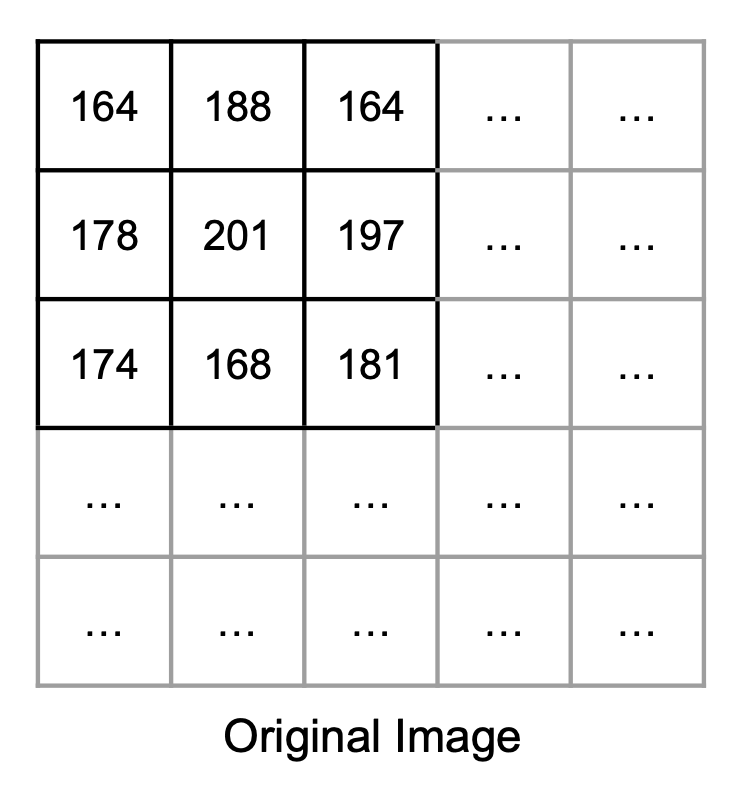Figure 5: The original image, the kernel, and the pre-allocated output image.

Observe that we are using a normalized kernel, i.e. its sum is 1. If our kernel was not normalized, consecutive convolutions would make the image darker/lighter overall, which isn't what we want. We can normalize a kernel by dividing by the sum of its elements, and you will need account for this in Project 2: Filter.

Also note that, as this kernel is symmetric, it is equal to its flipped self. That means we can make one fewer diagram :)

Next, we can proceed to iterate over every pixel in the output image to determine their values. For this example, we will look only at pixel (1, 1), the one with intensity 201 in the original image.

As described earlier, a region around the pixel is multiplied element-wise with the kernel, then summed:

Expand for full equation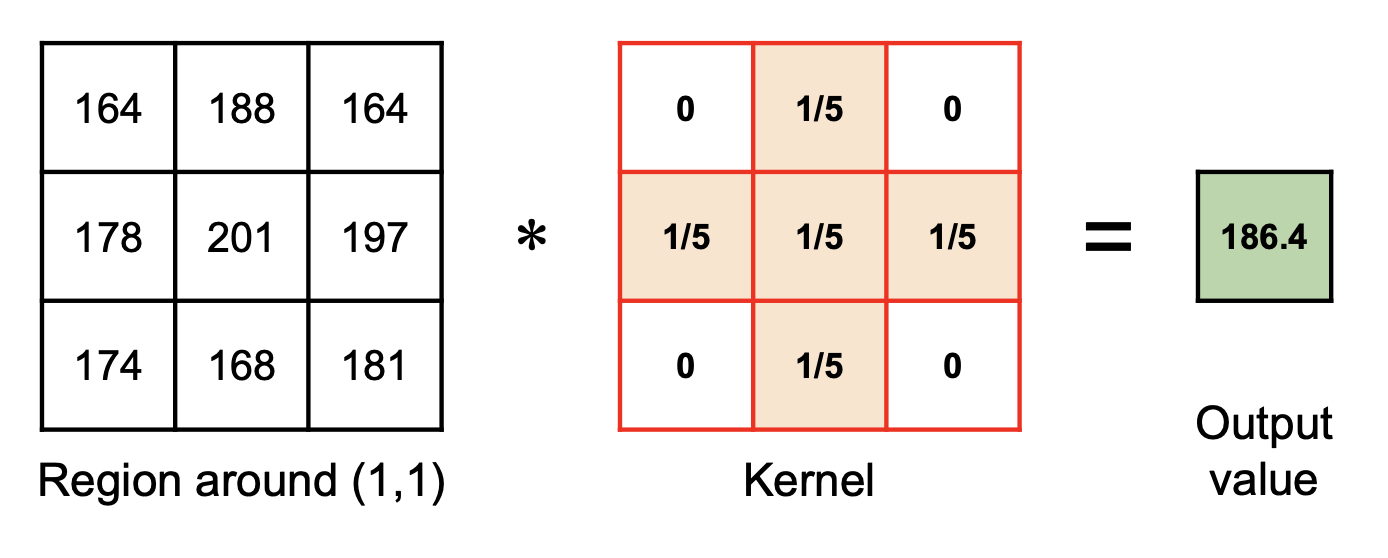Figure 6: A region centered on some pixel is multiplied element-wise with a kernel, then summed.

This sum is then stored as the pixel intensity in the output image:

This process of element-wise multiplication and summation must be repeated for every pixel in the output image. As you can probably guess, this makes convolution computationally expensive, due to the large number of multiplications, especially with larger kernels.

Extra: can we do it faster?

If the kernel is separable, then we can reduce computational cost significantly. Given an image and a kernel, "separating the filter" reduces our time complexity from to .

You will implement separable kernels for in Project 2: Filter. For this lab, however, we will only cover convolution with 2D kernels.

You may be wondering how to perform the above computation for pixels (0, 0), since the "region around it" exceeds the canvas bounds. We'll address that soon!

### Implementing Convolution

Finally, let's get to actually implementing the convolve2D() function in filterutils.cpp!

We will follow the steps outlined above, with the caveat that instead of actually flipping our kernel, we'll cleverly index into it "backwards", in a way that effectively flips it. This will be done as part of task 8.

First, let's prepare the output image.

In filterutils::convolve2D(), initialize a result RGBA vector to store your output image. This vector should have the same size as your input image, which is is stored in data.

Later, in order to perform our element-wise multiplication, we'll need to iterate through the kernel. So, let's also determine the kernel's dimensions.

Obtain the side length of kernel, and store it. You can assume that all kernels are square and have an odd number of rows and columns (so that they have a "center"), at least for this lab.

#### Beginning The Loop

Now, it's time to implement our element-wise multiplication and sum. This is a pretty big task, so don't be afraid to ask a TA or your peers for help!

1. Initialize redAcc, greenAcc, and blueAcc float variables to store the accumulated color channels.
2. Iterate over each element of the kernel, using the kernel dimensions you stored earlier.
• Remember that you must "flip" the kernel.

Tip: The convention for indexing we've been using starts (0, 0) in the top left corner moving right and down. If we flip the kernel, which corner do we begin and what directions do we move in? This should be a very small change to your code.

1. For each iteration, update redAcc, greenAcc, and blueAcc. Remember, they are the accumulated sum of the (RGBA pixel value) * (the corresponding kernel value). Recall that RGBA stores channel information as integers. The kernel however, is defined by floats. You will need to convert the pixel data to a float before applying the kernel's value to it.
• You might want to check that your pixel coordinates are within the image's bounds. The next task will address this.

#### Out-Of-Bounds Pixels

As promised earlier, we'll now discuss how to account for the case where the kernel extends beyond the boundary of the image, where pixel data is not defined. How can we obtain pixel "data" for, say, a pixel at (-1, -1)?

There are several ways to deal with this, and in filterutils.cpp, we have provided you some functions for this purpose:

1. getPixelRepeated()
// Repeats the pixel on the edge of the image such that A,B,C,D looks like ...A,A,A,B,C,D,D,D...
RGBA getPixelRepeated(std::vector<RGBA> &data, int width, int height, int x, int y) {
int newX = (x < 0) ? 0 : std::min(x, width  - 1);
int newY = (y < 0) ? 0 : std::min(y, height - 1);
return data[width * newY + newX];
}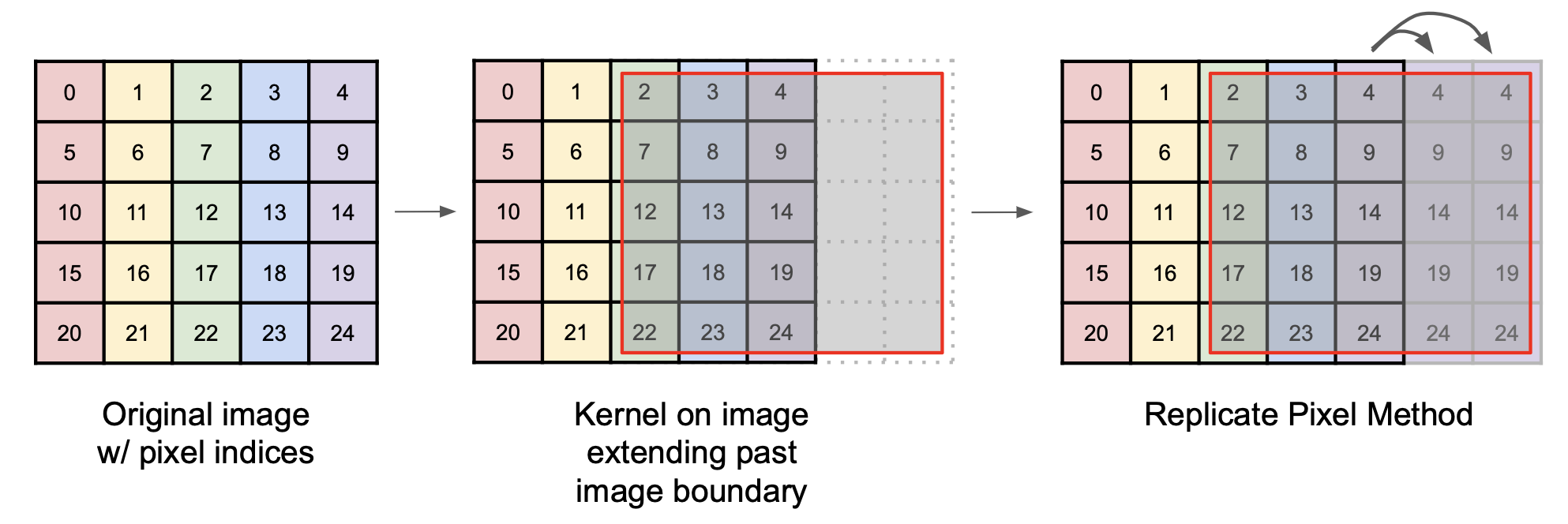Figure 8: The "repeat" method extends the pixel values at the edges of the image.
1. getPixelReflected()
// Flips the edge of the image such that A,B,C,D looks like ...C,B,A,B,C,D,C,B...
RGBA getPixelReflected(std::vector<RGBA> &data, int width, int height, int x, int y) {
// Task 9: implement this function

return RGBA{0, 0, 0, 255};
}

1. getPixelWrapped()
// Wraps the image such that A,B,C,D looks like ...C,D,A,B,C,D,A,B...
RGBA getPixelWrapped(std::vector<RGBA> &data, int width, int height, int x, int y) {
int newX = (x < 0) ? x + width  : x % width;
int newY = (y < 0) ? y + height : y % height;
return data[width * newY + newX];
}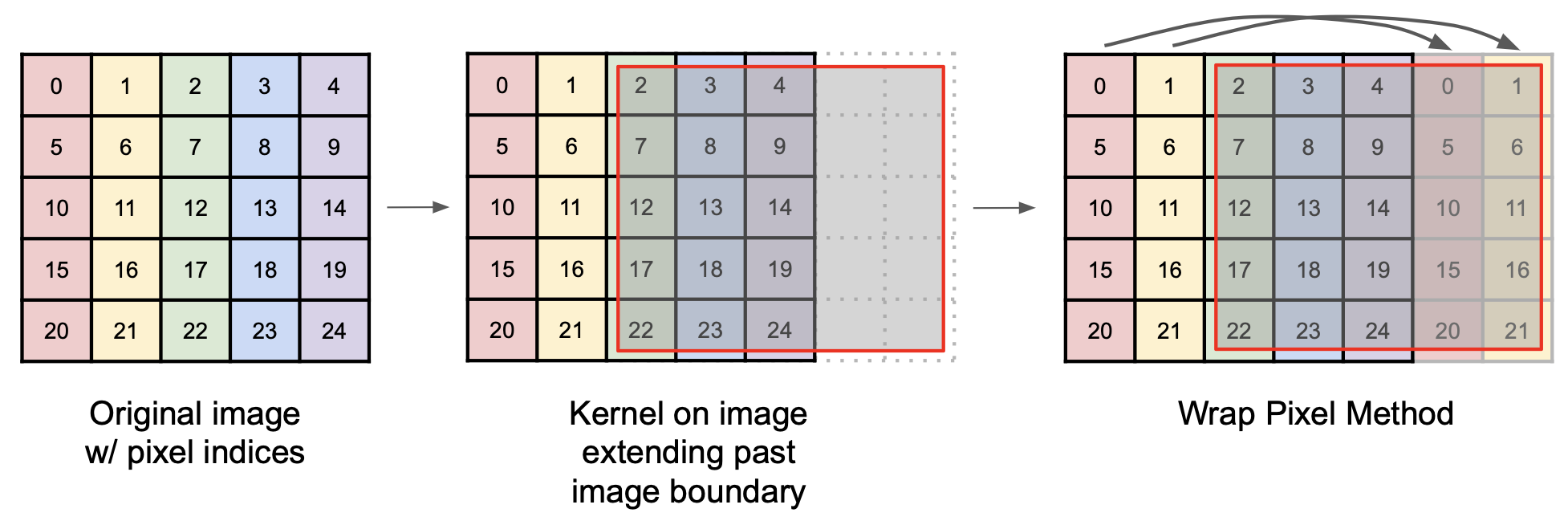Figure 10: The "wrap" method wraps the image around its edges.

We have implemented functions (1) getPixelRepeated() and (3) getPixelWrapped() for you, and you are welcome to use either one of them in convolve2D().

Implement function (2) getPixelReflected(), and verify that it works.

Having accumulated your red, green, and blue values, you now need to update result with the new RGBA value.

Using redAcc, greenAcc, and blueAcc, update the result vector.

• You may find the floatToUint8() utility function useful.
• We only care about fully-opaque images, so you can set the alpha value to 255.

#### Wrapping Up

Your result vector should now contain the output image's RGBA values. To display this image, we must overwrite the image data currently stored in data:

Copy the result vector into data. You may use a simple for loop, or std::copy (though this involves some knowledge of iterators).

Good work! You're done with the hardest part of this lab, and, in following sections, we'll use the convolve2D function you just implemented to perform some basic filtering. We will do so by defining different kernels for each filter, then convolving our input image with those kernels.

## Identity Filter

An identity filter convolves the input image with an identity kernel, returning the original image.

In filterIdentity(), initialize an identity kernel. You will have to figure out what this identity kernel is by yourself!

• The kernel should be a std::vector<float>.
• The side length of the kernel can be any odd number.
• While technically correct, please don't create a 1 x 1 kernel.
• Remember that if the kernel is too large, convolution can be very slow.

In the same function, call FilterUtils::convolve2D(). You will need to pass in the kernel you made in task 13 and the relevant canvas data (found in canvas2d.h). convolve2D() will convolve your image with the identity kernel.

Running and Testing:

Pass in identity as your command line argument.

Your filter should produce the following output:

## Shift Filter

A shift filter shifts the image by some number of pixels.

In filterShift(), initialize a kernel which, when convolved with an image, shifts the image one pixel to the left or to the right, depending on the value of shiftDir.

Optional task: implement createShiftKernel()

This function should create a shift kernel that is able to shift the image num pixels in the direction of shiftDir.

Call convolve2D(), passing in your kernel and appropriate canvas information. convolve2D() will convolve your image with the shift kernel.

Running and Testing:

Pass in either shiftLeft or shiftRight as your command line argument.

Your filter should produce the following output:Figure 12: A left-shift filter applied many times to an image, using the getPixelRepeated() approach.Figure 13: A left-shift filter applied many times to an image, using the getPixelWrapped() approach.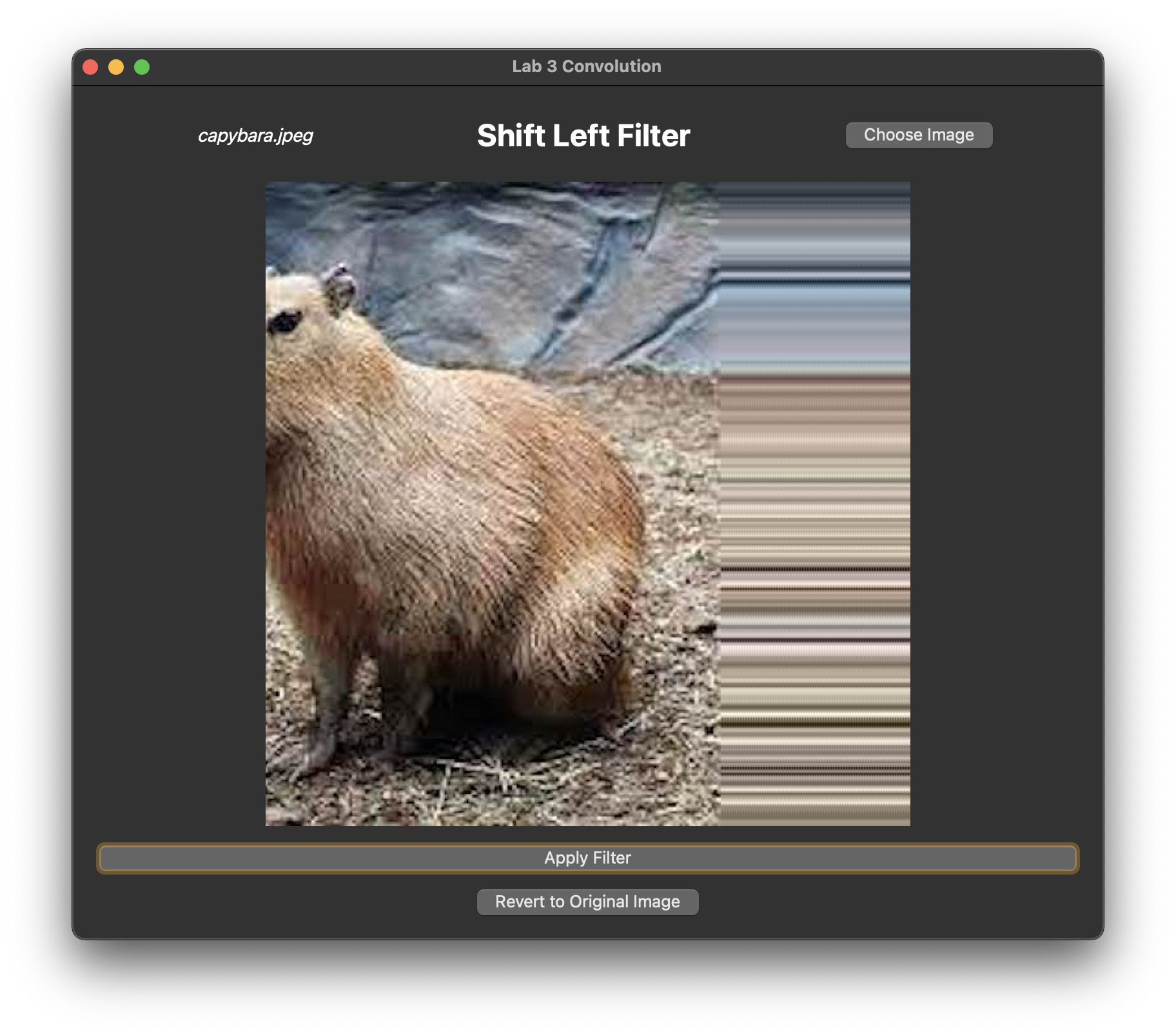Figure 14: A left-shift filter applied many times to an image, using the getPixelReflected() approach.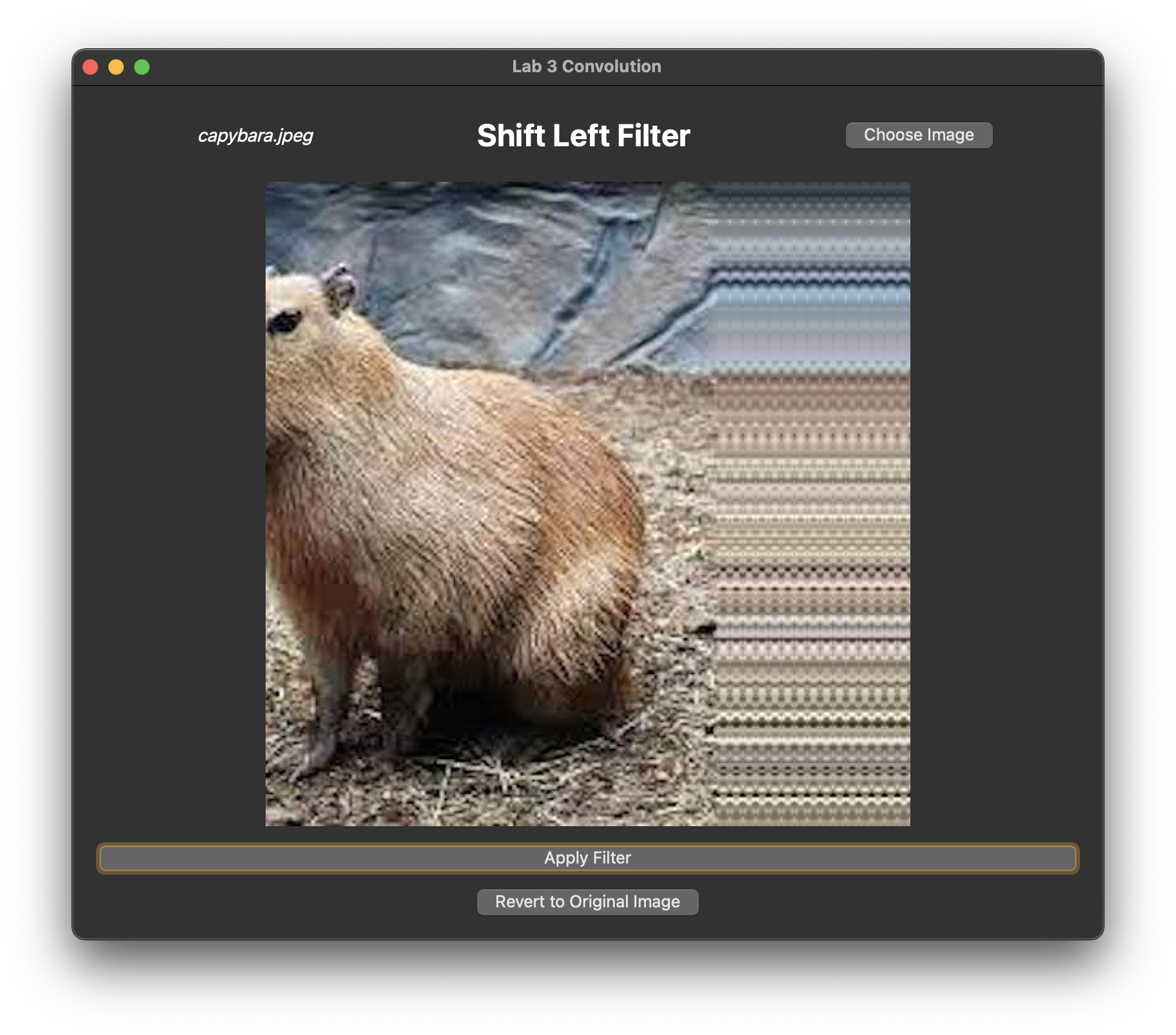Figure 15: A left-shift filter applied many times to an image, using the getPixelRepeated() approach. This one shifts the image by 5px at a time.

## End

Congrats on finishing the Convolution lab! Now, it's time to submit your code and get checked off by a TA. Be prepared to show the TA your working grayscale, invert, identity, shiftLeft, and shiftRight filters.

## Submission

Submit your Github repo for this lab to the "Lab 3: Convolution" assignment on Gradescope, then get checked off by a TA during lab hours.